Network Processor Module

# npm

## logml

2.1.2 • Public • Published

# Logml

Logml is a logical modelling framework based on the Boolean network theory where classic Boolean operators are replaced with fuzzy operators and where edges are tuned in order to adapt the speed and the strength of the signal that they transmit.

This tool is an adaptation of a methodology developed at Novadiscovery and freely accessible at:

Poret, A., Monteiro Sousa, C., Boissel, J.-P.: Enhancing boolean networks with fuzzy operators and edge tuning.arXiv:1407.1135 (2014)

## Installation

### Requirements

#### Fortran

For Linux, install gfortran

For OSX, Check that `gcc` (that comes with gfortran) has been correctly installed. If not, install it via brew:

#### NodeJS

Just install the last stable version from https://nodejs.org, or use Node Version Manager

#### Logml

To install Logml, just run the following command :

Now you can use the `logml` command:

## Usage

The Logml modelling and simulation tool takes a logical graph (.graphml format) as input. The tool will automatically parse the graph and generate the corresponding code. The logical model is then executed and results are plotted via a dedicated web interface.

### Logical graph

A logical graph is a special type of diagramatic structure in which entities are linked by logical relations symbolized by the logic operators: `AND`, `OR` and `NOT`.

We use for that the yED graph editor (which is freely available and runs on all major platforms), and the following graphical ontology: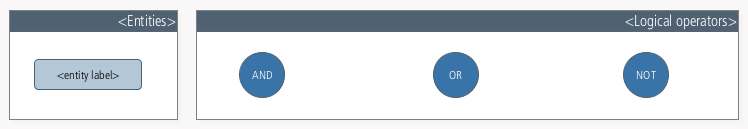Please find bellow an example of a logical graph, which will be used throughout this tutorial: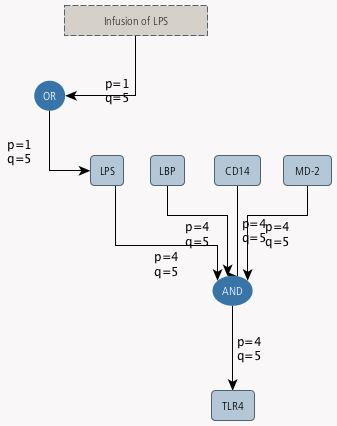In order to ensure the proper functioning of Logml tool, the following instructions must be observed carefully during the creation of the logical graph in yED.

#### Annotation of nodes

Each node of the graph (entity) need to be annotated (in yED, right click on the node > `properties` > `data`) with its qualitative initial values (integers only, and between 0 and 5). The node initial states are defined as following:

Annotation Meaning
`v0=5` full
`v0=4` much more
`v0=3` much
`v0=2` few
`v0=1` fewer
`v0=0` none

Please find below an example of node annotation: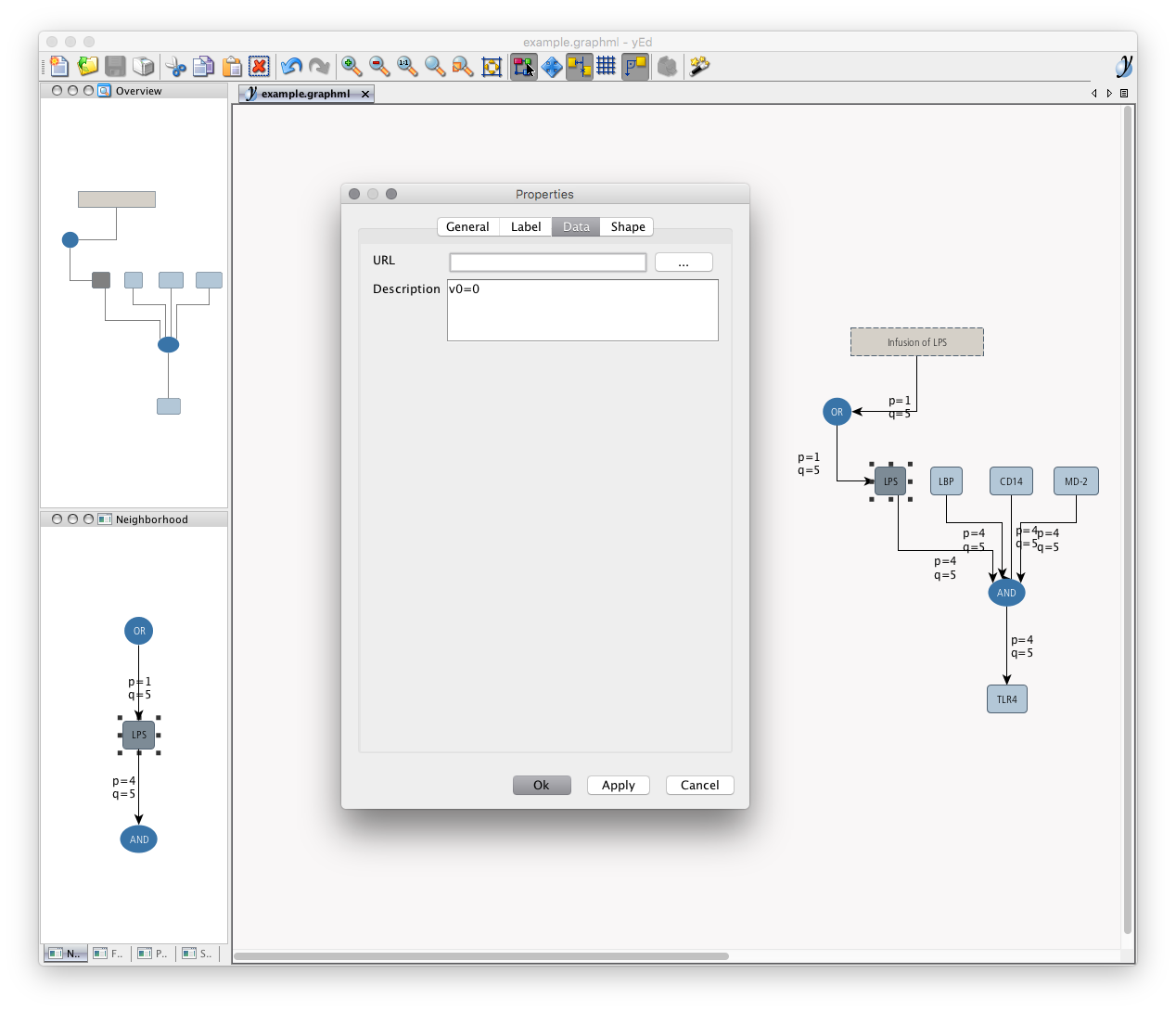#### Annotation of edges

Each edge need to be annotated (in yED, right click on the edge > `properties` > `general`) with its qualitative `p` and `q` values (integers only, and between 0 and 5).

• For each edges, `p` is the portion of its value which is updated at each iteration:
Annotation Meaning
`p=5` instantaneous
`p=4` faster
`p=3` fast
`p=2` slow
`p=1` slower
`p=0` down
• For each edges, `q` is the weakening of its value at each iteration:
Annotation Meaning
`q=5` strong
`q=4` weaker
`q=3` weak
`q=2` faint
`q=1` fainter
`q=0` down

Please find below an example of edge annotation: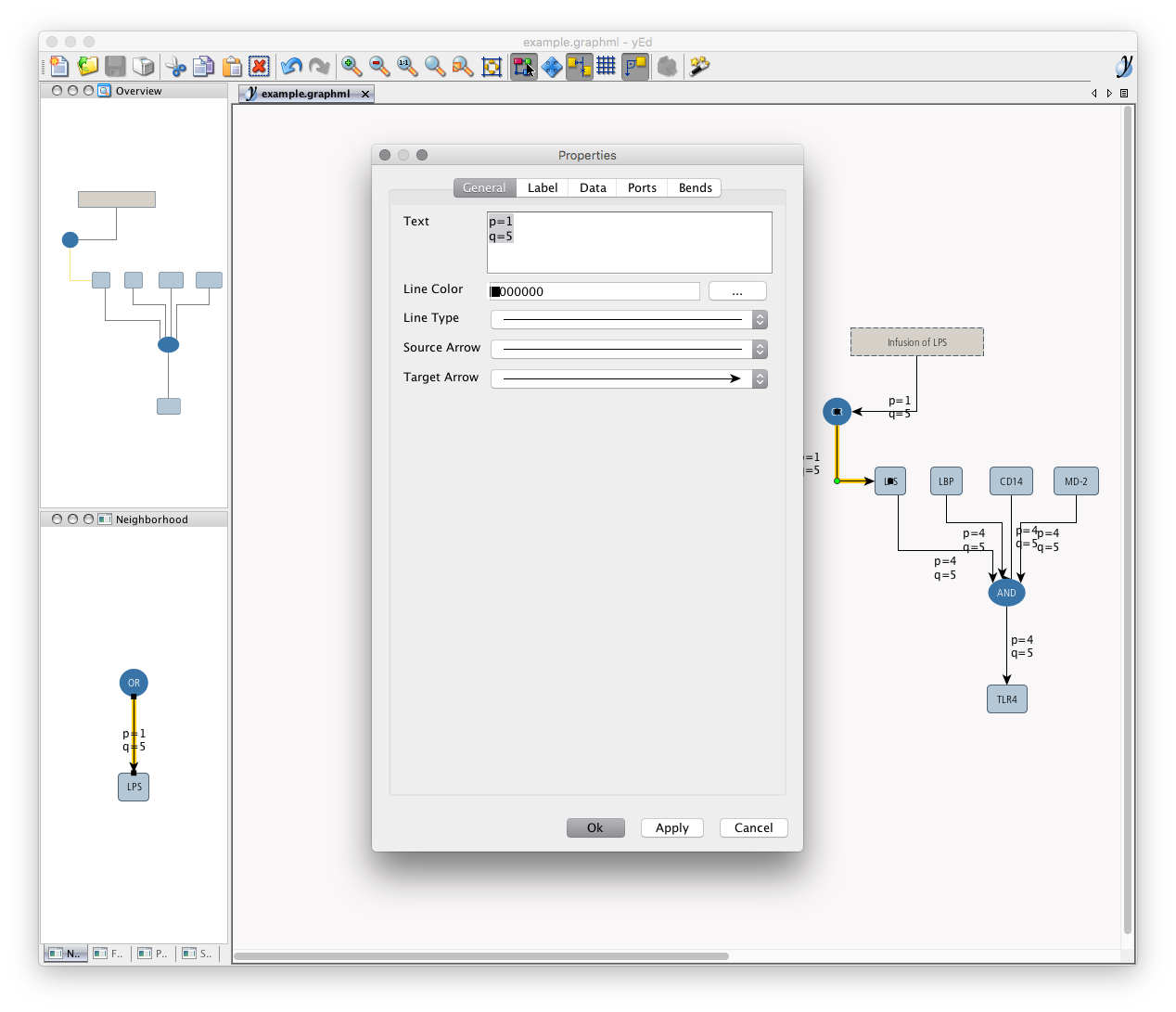#### Annotation of perturbed nodes

We can identify nodes that will be the subject of a disturbance by annotating (in yED, right click on the entity > `properties` > `data`) with:

• the strength of the disturbance applied to the node. The disturbed states will be defined as following (integers only, and between 0 and 5):
Annotation Meaning
`pwd=5` full
`pwd=4` much more
`pwd=3` much
`pwd=2` few
`pwd=1` fewer
`pwd=0` none
• the interval of iterations at which disturbances begin and end respectively. The intervals will be defined as following (integers only, and between 0 and 100):

`int=20,60`: in this case, perturbations will start after 20% of iterations, and will stop after 60% of iterations.

An example of disturbance annotation is presented in the following screenshot: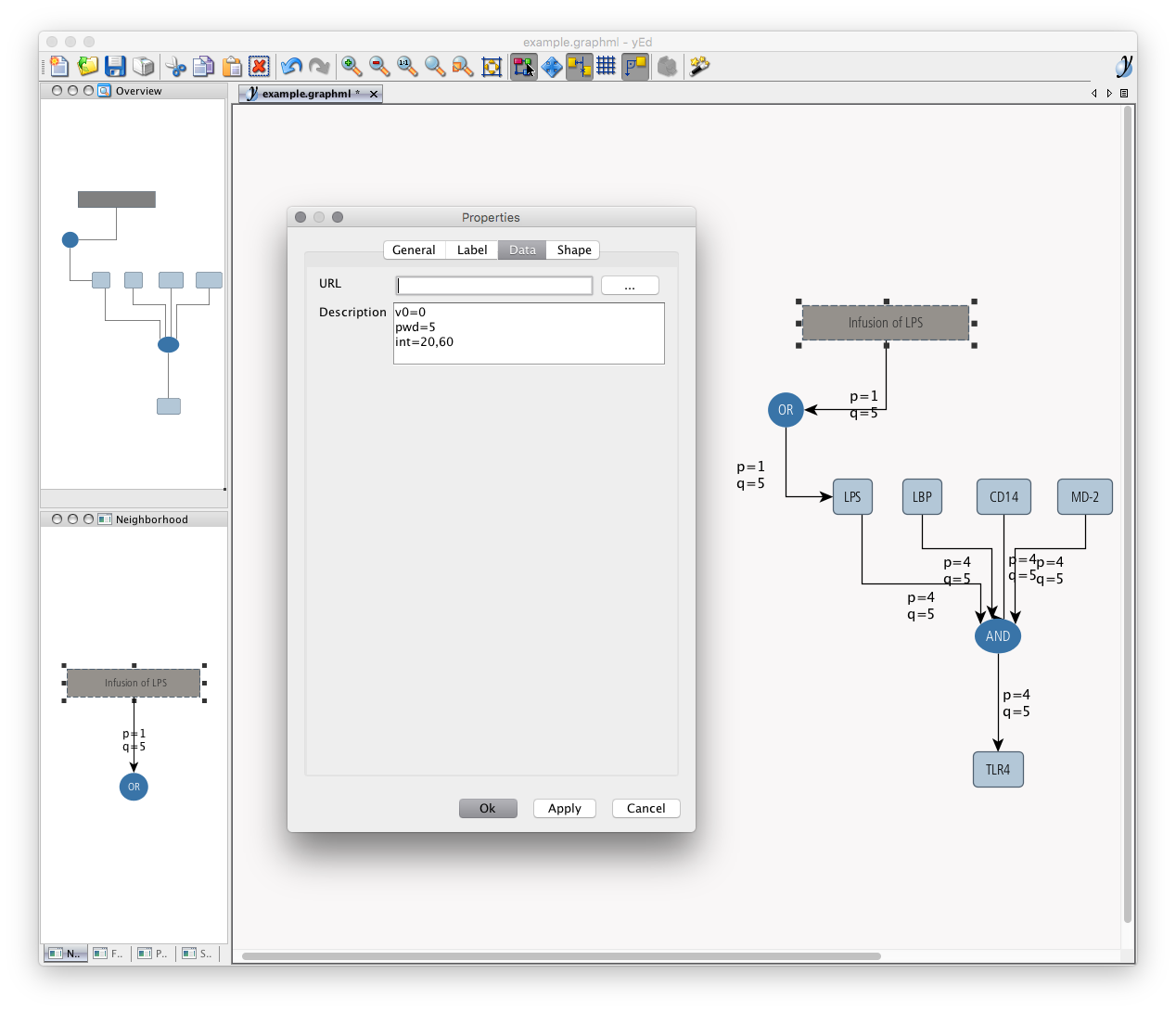## Example

We used the following Logml command with the `example.graphml`:

And we obtained the following output result: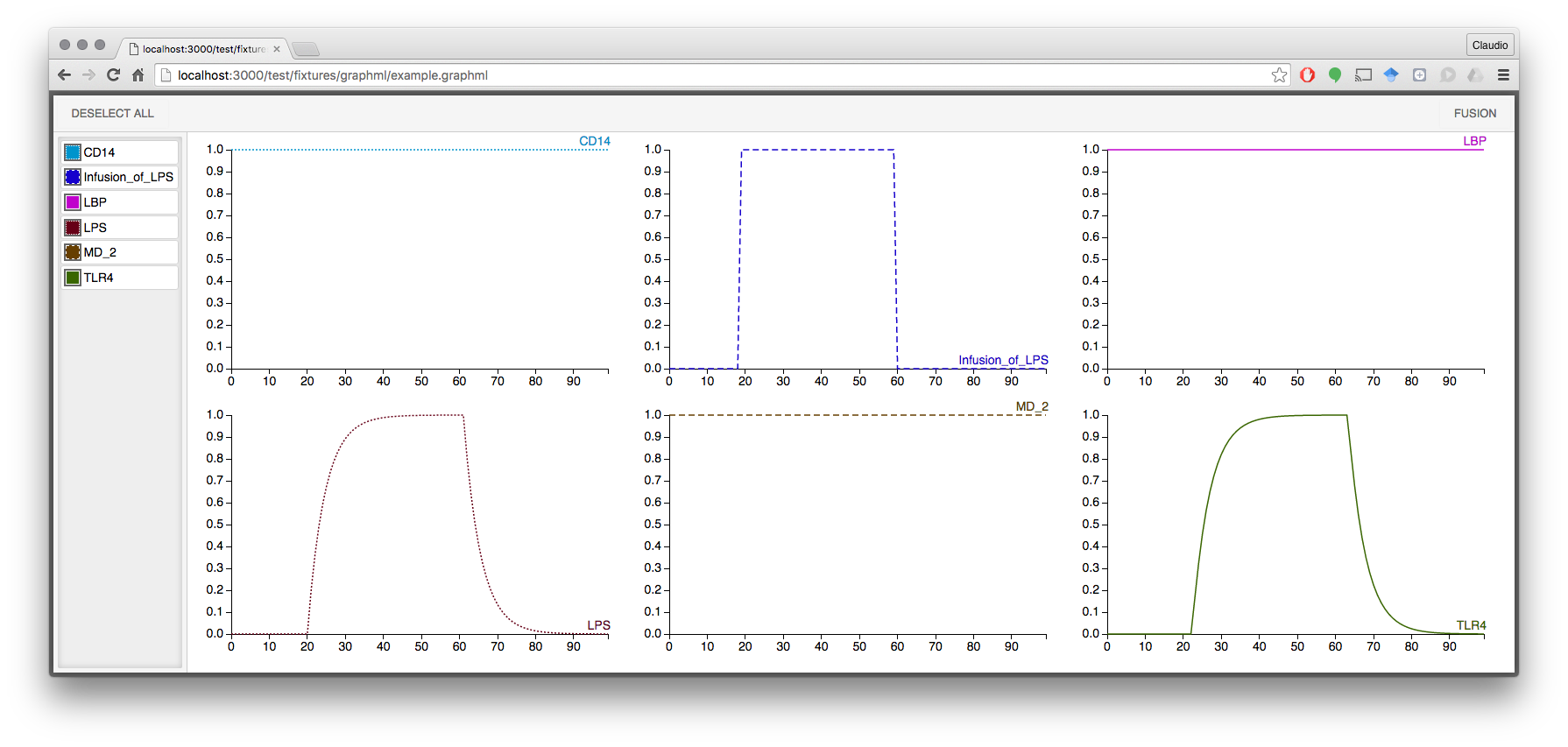## Keywords

### Install

`npm i logml`

### Repository

github.com/nodys/logml